# Hyperbola

(diff) ← Older revision | Latest revision (diff) | Newer revision → (diff)

A planar curve which is obtained as the intersection of a circular cone with a plane not passing through the apex of the cone and intersecting both its sheets. A hyperbola is the set of pointsof the surface (see Fig.) for which the modulus of the difference of the distance to two given points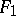and(the foci of the hyperbola) is constant and equal to. The distance between the foci of the hyperbola, known as its focal distance, is denoted by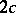.Figure: h048230a

The midpoint of the segmentis known as the centre of the hyperbola. The straight line including the location of the foci of the hyperbola is said to be the real (or focal) axis of the hyperbola. The straight line through the centre of the hyperbola perpendicular to the real axis is called the imaginary axis of the hyperbola. The imaginary and real axes of the hyperbola are its axes of symmetry. The number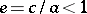is said to be its eccentricity. A diameter of a hyperbola is any straight line passing through its centre. Midpoints of parallel chords of a hyperbola lie on a diameter of it. The directrix of a hyperbola corresponding to a given focusis the straight line, perpendicular to the real axis, whose distance from the centre is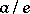and which lies on the same side of the centre as. A hyperbola has two directrices; it also has two asymptotes:where.

A hyperbola is a second-order curve. Its canonical equation has the form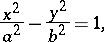whereandare the semi-axes of the hyperbola whileandare the running coordinates. The equation of the tangent to the hyperbola at a point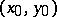is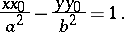The focal parameter of a hyperbola (the half-length of the chord passing through the focus perpendicularly to the focal axis of the hyperbola) is equal to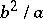. The equation of a hyperbola may be written in terms of the focal parameteraswhere,are polar coordinates and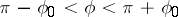, where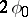is the angle between the asymptotes.

If, the hyperbola is called equilateral. The asymptotes of an equilateral hyperbola are mutually perpendicular; if they are taken as the coordinate axes, then the equation of an equilateral hyperbola is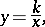i.e. an equilateral hyperbola is the graph of an inversely-proportional relationship.